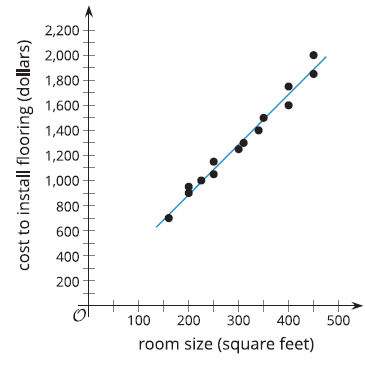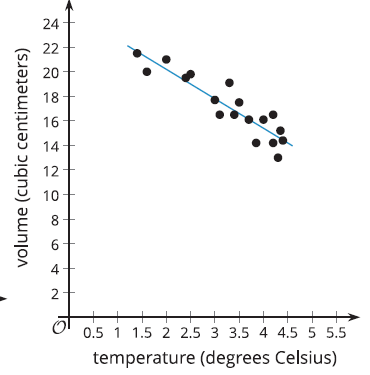# A.3.4.4 The Slope is the Thing

y = 4x + 87a. Using the horizontal axis for x and the vertical axis for y, interpret the slope of the linear model in the situation shown in the scatter plot. b. If the linear relationship continues to hold for the situation, interpret the y-intercept of the linear model in the situation provided.

y = -2.4x + 25a. Using the horizontal axis for x and the vertical axis for y, interpret the slope of the linear model in the situation shown in the scatter plot. b. If the linear relationship continues to hold for the situation, interpret the y-intercept of the linear model in the situation provided.